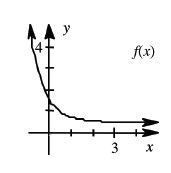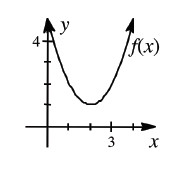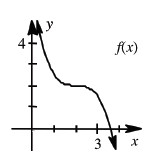### Home > CALC > Chapter 3 > Lesson 3.4.2 > Problem3-162

3-162.

For each graph below:

1. Trace $f(x)$ on your paper and write a slope statement for $f(x)$.

2. Sketch the graph of $f^\prime(x)$ using a different color.

1.1.1.Use your slope statements to sketch the derivative graphs. Pay attention to whether the slope has positive or negative values. Also pay attention to whether those values are increasing or decreasing.

Slope statements should tell a story, starting at the left side of the graph and ending at the right side. There should be a description of where the function is increasing or decreasing and how the rate at which it is increasing or decreasing is changing.